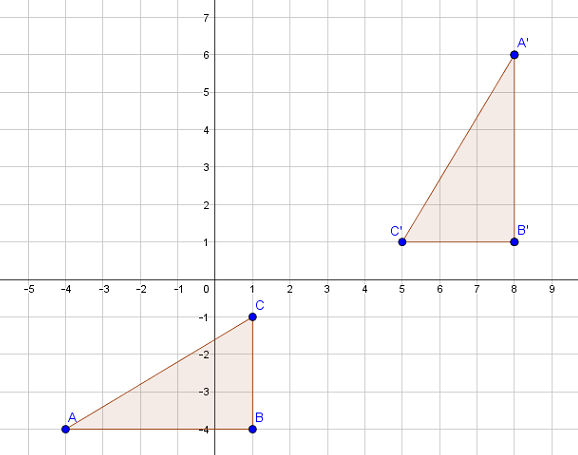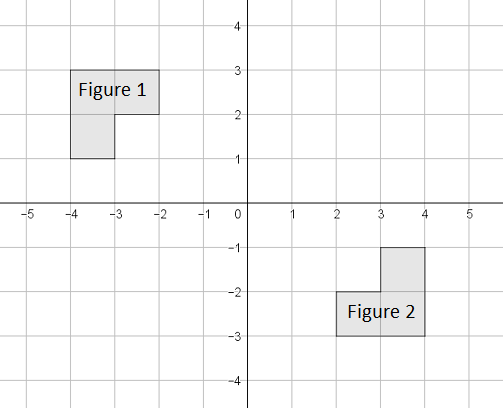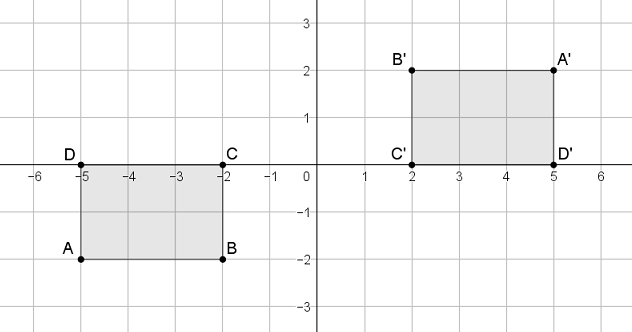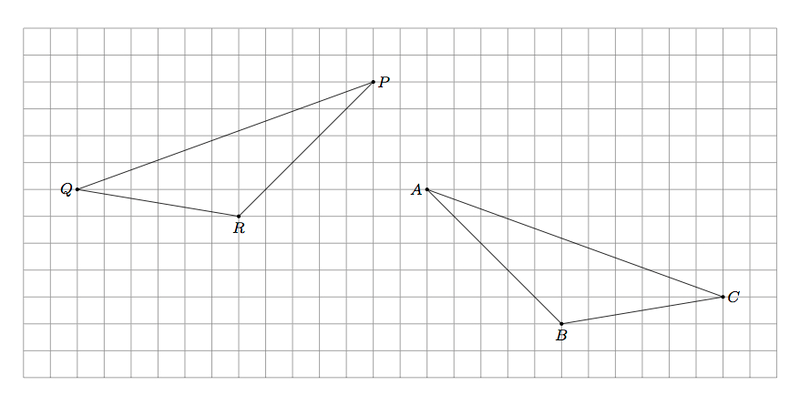Match Fishtank is now Fishtank Learning!

# Transformations and Angle Relationships

## Objective

Describe a sequence of rigid transformations that will map one figure onto another.

## Common Core Standards

### Core Standards

?

• 8.G.A.1.A — Lines are taken to lines, and line segments to line segments of the same length.

• 8.G.A.1.B — Angles are taken to angles of the same measure.

• 8.G.A.1.C — Parallel lines are taken to parallel lines.

• 8.G.A.2 — Understand that a two-dimensional figure is congruent to another if the second can be obtained from the first by a sequence of rotations, reflections, and translations; given two congruent figures, describe a sequence that exhibits the congruence between them.

?

• 4.MD.C.6

## Criteria for Success

?

1. Accurately describe, with precision, a sequence of transformations that demonstrates congruency between two figures (MP.6).

## Tips for Teachers

?

• In this lesson, students use any/all of the rigid transformations to map one figure to another to determine if they are congruent.
• Students can continue to use patty paper to test out and verify transformations, especially when working with reflections and rotations.
• The following materials are useful for this lesson: patty paper (or transparency paper) and graph paper.

#### Remote Learning Guidance

If you need to adapt or shorten this lesson for remote learning, we suggest prioritizing Anchor Problem 1 (benefits from worked example) and Anchor Problem 2 (benefits from discussion) . Find more guidance on adapting our math curriculum for remote learning here.

#### Fishtank Plus

• Problem Set
• Student Handout Editor
• Vocabulary Package

## Anchor Problems

?

### Problem 1

Triangle ${{ABC}}$ has been moved according to the following sequence: a translation followed by a rotation followed by a reflection.

With precision, describe each rigid motion that would map triangle ${{ABC}}$ onto triangle ${A'B'C'}$.#### References

EngageNY Mathematics Grade 8 Mathematics > Module 2 > Topic B > Lesson 10Teacher Version: Exit Ticket

Grade 8 Mathematics > Module 2 > Topic B > Lesson 10 of the New York State Common Core Mathematics Curriculum from EngageNY and Great Minds. © 2015 Great Minds. Licensed by EngageNY of the New York State Education Department under the CC BY-NC-SA 3.0 US license. Accessed Dec. 2, 2016, 5:15 p.m..

Modified by Fishtank Learning, Inc.

### Problem 2

Avik believes that Figure 1 and Figure 2, shown below, are congruent because he can use two reflections to map one figure to the other.

Sonya also believes the two figures are congruent because she can use a single rotation to map one figure to the other.Are both Avik and Sonya right? Explain with precision the transformation(s) that will show the two figures are congruent.

### Problem 3

Rectangle ${{ABCD}}$ and ${{A'B'C'D'}}$ are shown below.Which sequence of transformations show that rectangle ${{ABCD}}$ and rectangle ${{A'B'C'D'}}$ are congruent? Select all that apply.

1. A translation 7 units right and 2 units up
2. A reflection over the $x-$axis followed by a reflection over the $y-$axis
3. A reflection over the $x-$axis followed by a translation 7 units to the right
4. A rotation of 90° clockwise
5. A rotation of 180° clockwise

## Problem Set

?The following resources include problems and activities aligned to the objective of the lesson that can be used to create your own problem set.

• Multiple-choice options are good problems to engage students in evaluating multiple possible answer choices. Examples to include:
• Given transformed figures, select the correct description of the transformation sequence.
• Given a description of a transformation sequence, select the correct visual representation.
• Multiple-select items about properties of transformations where there is more than one correct answer
• Error analysis of incorrect descriptions of transformation sequences and/or transformations performed incorrectly
• Examples should include a variety of combinations of transformations such as rotations and rotations, translations and rotations, all three, reflection, and 180° rotation.

?

The two triangles in the picture below are congruent:a.     Give a sequence of rotations, translations, and/or reflections that take ${\triangle PRQ}$ to ${\triangle ABC}$.

b.     Is it possible to show the congruence in part (a) using only translations and rotations? Explain.

#### References

Illustrative Mathematics Congruent Triangles

Congruent Triangles, accessed on Oct. 13, 2017, 4:04 p.m., is licensed by Illustrative Mathematics under either the CC BY 4.0 or CC BY-NC-SA 4.0. For further information, contact Illustrative Mathematics.

?